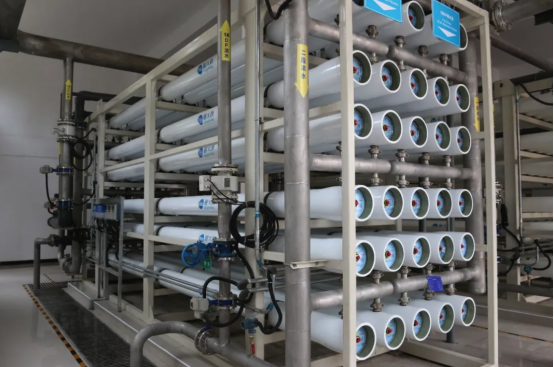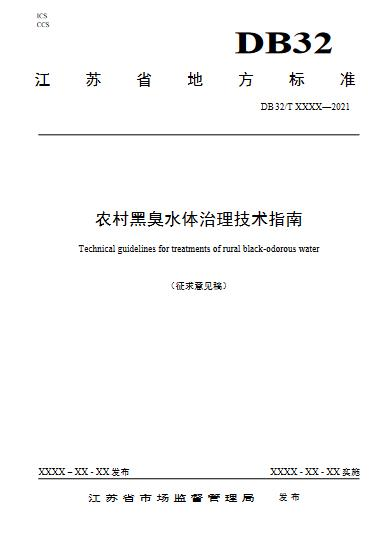• 企业 产品 信息

# 为什么CNP比控制在100：5：1？

1、CNP比100：5：1的比例是针对于好氧除碳工艺的营养比！而非厌氧与脱氮工艺的CNP比！

2、碳氮磷代表的值，Ｃ目前争议最多，代表COD或者BOD各有支持人群（其实工程应用比较灵活，多了少加，少了多加，营养源的计算只是一个大体的估值,笔者颜胖子个人偏重于COD的说法，这样的计算结果不会使碳源投加过量，毕竟一切服务于实际）；N一般指总凯氏氮（TKN），包括有机氮和氨氮，但不包括亚硝氮和硝态氮；而磷最没有争议性一般为正磷酸盐。

3、100：5：1比例的来源：

1、外部碳源投加量简易计算方法

Cm=20N-C （式1）

Cm—必须投加的外部碳源量（以COD计）mg/l； 20—CN比； N—需要去除的TKN的量，mg/l

C—进出水的碳源差值（以COD计）mg/l

N=Ne-Ns （式2）

Ne—进水实际TKN浓度mg/l； Ns—二沉池TKN排放指标mg/l

C=Ce-Cs （式3）

Ce—进水实际COD浓度mg/l； Cs—二沉池COD排放指标mg/l

2、案例计算

N=Ne-Ns=15-5=10(mgN／L)

C=Ce-Cs=100-10=90mg／L

Cm=20N-C=20×10-90=110(mgCOD/L)

Cd=QCm=1×10^4×110×10^-3=1100(kgCOD/d)

1、外部碳源投加量简易计算方法

Cm=5N （式4）

Cm—必须投加的外部碳源量（以COD计）mg/l； 5—反硝化1kgNO-3-N需投加外部碳源(以COD计)5kg； N—需要外部碳源去除的TN量，mg/l

N=Ne-Ns （式5）

Ne—二沉池出水实际TN浓度mg/l； Ns—二沉池TN排放标准mg/l

2、案例计算：

N=Ne-Ns=20-15=5(mgN／L)

Cm=5N=5×5=25(mgCOD/L)

Cd=QCm=1×10^4×25×10^-3=250(kgCOD/d)

1、外部碳源投加量简易计算方法

Cm=15P-C （式6）

Cm—必须投加的外部碳源量（以COD计）mg/l； 15—CP比； P—需要外部碳源去除的TP量，mg/l

C—进出水的碳源差值（以COD计）mg/l

2、需用外部碳源去除的TP量计算

P=Pe-Ps （式7）

Pe—进水TP浓度mg/l；Ps—二沉池TP排放标准mg/l

C=Ce-Cs （式8）

Ce—进水实际COD浓度mg/l； Cs—二沉池COD排放标准mg/l

2、案例计算：

P=Pe-Ps=11-1=10(mgN／L)

C=Ce-Cs=100-10=90mg／L

Cm=15P-C=15×10-90=60(mgCOD/L)

Cd=QCm=1×10^4×60×10^-3=600(kgCOD/d)2021年最新全国拟在建污水处理项目汇总